## 1 Introduction

Futures extend the actor programming model (This paper focuses on futures. From this perspective we consider the actor-, task-, and active object-based models as synonymous.) to express call-return synchronisation of message sends . Each actor is single-threaded, but different actors execute concurrently. Communication between actors happens via asynchronous method calls (messages), which immediately return a future; futures are placeholders for the eventual result of these asynchronous method calls. An actor processes one message at a time and each message has associated a future that will be fulfilled with the returned value of the method. Futures are first-class values, and operations on them may be blocking, such as getting the result out of the future ($$\mathtt {get}$$), or asynchronous, such as attaching a callback to a future. This last operation, known as future chaining (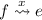), attaches a closure $$\lambda x.e$$ to the future f and immediately returns a new future that will contain the result of applying the closure to the value eventually stored in future f.

Consider the following code (in the actor-based language Encore (https://github.com/parapluu/encore) ) that implements the broker delegation pattern: the developer’s intention is to connect clients (the callers of the $$\mathtt{Broker}$$ actor) to a pool of actors that will actually process a job (lines 6–7):

The problem with this code is that the connection to the $$\mathtt{Broker}$$ cannot be completed immediately without blocking the $$\mathtt{Broker}$$’s thread of execution: returning the result of the worker running the computation requires that the $$\mathtt{Broker}$$ blocks until the future is fulfilled (line 8). This implementation makes the $$\mathtt{Broker}$$ the bottleneck of the application.

One obvious way to avoid this bottleneck is by returning the future, instead of blocking on it, as in the following code:

This solution removes the blocking from $$\mathtt{Broker}$$, but returns a future, which results in the client receiving a future containing a future $$\textit{Fut}~(\textit{Fut}~int)$$, cluttering client code and making the typing more complex.

Another way to avoid the bottleneck is to not block but yield the current thread until the future is fulfilled. This can be done using the $$\mathtt{await}$$ command [2, 3], which frees up the $$\mathtt{Broker}$$ to do other work:Footnote 1

This solution frees up the $$\mathtt{Broker}$$, but can result in a lot of memory being consumed to hold the waiting instances of calls $$\mathtt{Broker.run()}$$.

Another alternative is to use promises . A promise can be passed around and fulfilled explicitly at the point where the corresponding result is known. Passing a promise around is akin to passing the responsibility to provide a particular result, thereby fulfilling the promise.

Promises are problematic because they diverge from the commonplace call-return control flow, there is no explicit requirement to actually fulfil a promise, and care is required to avoid fulfilling multiple times. This latter issue, fulfilling a promise multiple times, can be solved by a substructural type system, which guarantees a single writer to the promise [5, 6]. Substructural type systems are more complex and not mainstream, which rules out adoption in languages such as Java and C#. Our solution relies on futures and is suitable for mainstream languages.

The main difference between promises and futures are that developers explicitly create and fulfil promises, whereas futures are implicitly created and fulfilled. Promises are thus more flexible at the expense of any fulfilment guarantees.

This paper explores a construct called forward that retains the guarantees of using futures, while allowing some degree of delegation of responsibility to fulfil a future, as in promises. This construct was first proposed a while ago , but only recently has been implemented in the language Encore .

With forward, the $$\mathtt{run}$$ of Broker method now becomes:

Forward delegates the fulfilment of the future that $$\mathtt{run}$$ will put its result in, to the call $$\mathtt{worker!start(job)}$$. Using forward frees up the $$\mathtt{Broker}$$ object, as $$\mathtt{run}$$ completes immediately, though the future is fulfilled only when $$\mathtt{worker!start(job)}$$ produces a result.

The paper makes the following contributions:

• a formalisation and soundness proof of the forward construct in a concise, high-level language (Sect. 2);

• a formalisation of a low-level, promise-based language (Sect. 3),

• a translation from the high-level language to the low-level language, a proof of program equivalence, between the high-level language and its translation to the low-level language (Sect. 4); and

• microbenchmarks that compare the $$\mathtt{get}$$-and-$$\mathtt{return}$$ and $$\mathtt{await}$$-and-$$\mathtt{get}$$ pattern versus the $$\mathtt{forward}$$ construct (Sect. 5).

## 2 A Core Calculus of Futures and Forward

This section presents a core calculus that includes tasks, futures and operations on them, and forward. The calculus consists of two levels: expressions and configurations. Expressions correspond to programs and what tasks evaluate. Configurations capture the run-time configuration; they are collections of tasks (running expressions), futures, and chains. This calculus is much more concise than the previous formalisation of forward .

The syntax of the core calculus is as follows: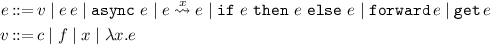Expressions include values (v), function application (e e), spawning asynchronous computations ($$\mathtt {async}~e$$), future chaining (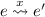), which attaches $$\lambda x.e'$$ onto a future to run as soon as the future produced by e is fulfilled, if-then-else expressions, $$\mathtt {forward}$$, and $$\mathtt {get}$$, which extracts the value from a future. Values are constants (c), futures (f), variables (x) and lambda abstractions ($$\lambda x.e$$). The calculus has neither actors nor message sends/method calls. For our purposes, tasks play the role of actors and spawning asynchronous computations is analogous to message sends.

Configurations, $$config _{}$$, give a partial view on the system and are (non-empty) multisets of tasks, futures and chains. They have the following syntax:

\begin{aligned} \textit{config}&\,{::=} \,( fut _{f} ) \, \mid \, ( fut _{ f }\ v ) \, \mid \, ( task _{ f } \ e) \, \mid \, ( chain _{f} \ f \ e) \, \mid \, config _{} \, config _{} \end{aligned}

Future configurations are $$( fut _{f} )$$ and $$( fut _{ f }\ v )$$, representing an unfulfilled future f and a fulfilled future f with value v. Configuration $$( task _{ f } \ e)$$ is a task running expression e that will write the result of e in future f.Footnote 2 Configuration $$( chain _{f} \ g \ e)$$ denotes a computation that waits until future g is fulfilled, applies expression e to the value stored in g in a new task whose result will be stored in future f.

The initial configuration for program e is $$( task _{ f } \ e) \ ( fut _{f} )$$, where the result of e will be written into future f at the end result of the program’s execution.

### 2.1 Operational Semantics

The operational semantics use a small-step semantics with reduction-based, contextual rules for evaluation within tasks. Evaluation contexts E contains a hole $$\bullet$$ that denotes where the next reduction step happens :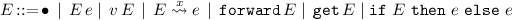The evaluation rules are given in Fig. 1. The evaluation of if-then-else expressions and functions applications proceed in the standard fashion (Red-If-True, Red-If-False, and Red-$$\beta$$). The async construct spawns a new task to execute the given expression, and creates a new future to store its result (Red-Async). When the spawned task finishes its execution, it places the value in the designated future (Red-Fut-Fulfil). To obtain the contents of a future, the blocking construct get stops the execution of the task until the future is fulfilled (Red-Get). Chaining an expression on a future results immediately in a new future that will eventually contain the result of evaluating the expression, and a chain configuration storing the expression is connected with the original future (Red-Chain-Create). When the future is fulfilled, any chain configurations become task configurations and start evaluating the stored expression on the value stored in the future (Red-Chain-Run). Forward applies to a future where the result of the future computation will be the result of the current computation, stored in the future associated with the current task. Forwarding to future h throws away the remainder of the body of the current task and chains the identity function on the future, the effect of which is to copy the eventual result stored in h into the current future (Red-Fwd-Fut).

The configuration evaluation rules (Fig. 2) describe how configurations make progress, which is either by some subconfiguration making progress, or by rewriting a configuration to one that will make progress using the equations of multisets.

Example and Optimisations. The following example illustrates some aspects of the calculus.

\begin{aligned}&( task _{ f } \ E[\mathtt {async}~ \ (\mathtt {forward} \, \ h)])\ ( fut _{ h }\ 42 ) \\&\xrightarrow {\textsc {Red-Async}} ~~ ( task _{ f } \ E[g])\ ( fut _{ h }\ 42 ) \ ( fut _{g} )\ ( task _{ g } \ \mathtt {forward} \, \ h) \\&\xrightarrow {\textsc {Red-Fwd-Fut}} ~~ ( task _{ f } \ E[g])\ ( fut _{ h }\ 42 ) \ ( fut _{g} )\ ( chain _{g} \ h \ \lambda x.x) \\&\xrightarrow {\textsc {Red-Chain-Run}} ~~( task _{ f } \ E[g])\ ( fut _{ h }\ 42 ) \ ( fut _{g} )\ ( task _{ g } \ (\lambda x.x)~42) \\&\xrightarrow {\textsc {Red-}\beta } ~~( task _{ f } \ E[g])\ ( fut _{ h }\ 42 ) \ ( fut _{g} )\ ( task _{ g } \ 42) \\&\xrightarrow {\textsc {Red-Fut-Fulfil}} ~~ ( task _{ f } \ E[g])\ ( fut _{ h }\ 42 ) \ ( fut _{ g }\ 42 ) \end{aligned}

Firstly, a new task is spawned with the use of $$\mathtt{async}$$. This task forwards the responsibility to fulfil its future to (the task fulfilling) future h, i.e. future g gets fulfilled with the value contained in future h.

Two special cases of $$\mathtt {forward}$$ can be given more direct reduction sequences, which correspond to optimisations performed in the Encore compiler. The first case corresponds to forwarding directly to another method call, which is the primary use case for $$\mathtt {forward}$$, namely, forwarding to another method $$\mathtt{forward(e!m())}$$. The optimised reduction rule is

$$( task _{ f } \ E[\mathtt {forward} \, (\mathtt {async}~ e)]) \rightarrow ( task _{ f } \ e)$$

For comparison, the standard reduction sequenceFootnote 3 is

\begin{aligned}&( task _{ f } \ E[\mathtt {forward} \, \ (\mathtt {async}~ e)]) \rightarrow ( task _{ f } \ E[\mathtt {forward} \, \ g])\ ( task _{ g } \ e)\ ( fut _{g} ) \\&\rightarrow ( chain _{f} \ g \ \lambda x.x)\ ( task _{ g } \ e)\ ( fut _{g} ) \rightarrow ^* ( chain _{f} \ g \ \lambda x.x)\ ( task _{ g } \ v)\ ( fut _{g} ) \\&\rightarrow ( chain _{f} \ g \ \lambda x.x)\ ( fut _{ g }\ v ) \rightarrow ( task _{ f } \ (\lambda x.x)~v)\ ( fut _{ g }\ v ) \rightarrow ( task _{ f } \ v)\ ( fut _{ g }\ v ) \end{aligned}

This can be seen as equivalent to the reduction sequence

$$( task _{ f } \ E[\mathtt {forward} \, (\mathtt {async}~ e)]) \rightarrow ( task _{ f } \ e) \rightarrow ^* ( task _{ f } \ v)$$

because the future g will no longer be accessible.

Similarly, forwarding a future chain can be reduced directly to a chain configuration: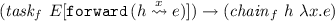In both cases, forward can be seen as making a call-with-current-future.

### 2.2 Static Semantics

The type system has basic types, K, and future types:

$$\tau \,{::=}\, K \mid \textit{Fut}~\tau$$

The typing rules (Fig. 3) define the judgement $$\varGamma \vdash _\rho e : \tau$$, which states that in the typing environment $$\varGamma$$, which gives the types of futures and free variables, expression e has type $$\tau$$, where $$\rho$$ is the expected task type, the result type of the task in which the expression appears. $$\rho$$ ranges over both types $$\tau$$ and symbol $$\bullet$$ which is not a type. $$\bullet$$ is used to prevent the use of $$\mathtt {forward}$$ in contexts where the expected task type is not clear, specifically within closures, as a closure can be passed between tasks and run in a context different from their defining contexts. The types of constants are assumed to be provided (Rule T-Constant). Variables and futures types are defined in the typing environment (Rules T-Variable and T-Future). Function application and abstraction have the standard typing rules (Rules T-Application and T-Abstraction), except that within the body of a closure the expected task type is not known. When $$\mathtt {async}$$ is applied to an expression e, a new task is created and the expected task type changes to the type of the expression. The result type of the $$\mathtt {async}$$ call is a future type of the expression’s type (Rule T-Async). Chaining is essentially mapping for the $$\textit{Fut}$$ type constructor, and rule T-Chain reflects this fact. In addition, because chaining ultimately creates a new task to run the expression, the expected task type $$\rho$$ changes to the return type of the expression. Getting the value from a future of some type results in a value of that type (Rule T-Get). Forwarding requires the argument to $$\mathtt {forward} \,$$ to be a future of the same type as the expected task type (Rule T-Forward). As $$\mathtt {forward} \,$$ does not return locally, the result type is arbitrary.

Well-formed configurations, $$\varGamma \vdash config _{} \, \textit{ok} {}$$, are typed against environment, $$\varGamma$$, that gives the types of futures (Fig. 4). The type rules depend on the following definitions.

### Definition 1

The function defs($$config _{}$$) extracts the set of futures present in a configuration $$config _{}$$.

\begin{aligned}&\textit{defs}(( fut _{f} )) \, =\, \textit{defs}(( fut _{ f }\ v ) ) \, =\, \{f\} \\&\textit{defs}(( config _{1} \, config _{2} )) \, =\, \textit{defs}( config _{1} ) \, \cup \, \textit{defs}( config _{2} ) \\&\textit{defs}(\_) \, =\, \varnothing \end{aligned}

### Definition 2

The function writers($$config _{}$$) extracts the set of writers to futures in configuration config.

\begin{aligned}&\textit{writers}(( chain _{f} \ g \ e)) \, =\, \textit{writers}(( task _{ f } \ e)) \, =\, \{f\} \\&\textit{writers}( config _{1} \, config _{2} ) \, =\, \textit{writers}( config _{1} ) \, \cup \, \textit{writers}( config _{2} ) \\&\textit{writers}(\_) \, =\, \varnothing \end{aligned}

Rules $$\textsc {Fut}$$ and $$\textsc {F-Fut}$$ define well-formed future configurations. Rules $$\textsc {Task}$$ and $$\textsc {Chain}$$ define well-formed task and future chaining configurations and set the expected task types. Rule $$\textsc {Config}$$ defines how to build larger configurations from smaller ones. Each future may be defined at most once and there is at most one writer to each future.

The rules for well-formed configurations apply to partial configurations. Complete configurations can be typed by adding extra conditions to ensure that all futures in $$\varGamma$$ have a future configuration, there is a one-to-one correspondence between tasks/chains and unfulfilled futures, and dependencies between tasks are acyclic. These definitions have been omitted and are similar to those found in our earlier work .

Formal Properties. The proof of soundness of the type system follows standard techniques . The proof of progress requires that there is no deadlock, which follows as there is no cyclic dependency between tasks .

### Lemma 1

(Type preservation). If $$\varGamma \vdash config {} \ \textit{ok} {}$$ and $$config {} \rightarrow config {'}$$, then there exists a $$\varGamma '$$ such that $$\varGamma ' \supset \varGamma$$ and $$\varGamma ' \vdash config {'} \ \textit{ok}$$.

### Proof

By induction on the derivation of $$config _{} \rightarrow config {'}$$.    $$\square$$

### Definition 3

(Terminal Configuration). A complete configuration $$config _{}$$ is terminal iff every element of the configuration has the shape: $$( fut _{ f }\ v )$$.

### Lemma 2

(Progress). For a complete configuration $$config _{}$$, if $$\varGamma \vdash config _{} \ \textit{ok}$$, then $$config {}$$ is a terminal configuration or there exists a $$config {'}$$ such that $$config _{} \rightarrow config {'}$$.

### Proof

By induction on a derivation of $$config _{} \rightarrow config {'}$$, relying on the invariance of the acyclicity of task dependencies.    $$\square$$

## 3 A Promising Implementation Calculus

The implementation of forward in the Encore programming language is via compilation into C, linking with Pony’s actor-based run-time . At this level, Encore’s futures are treated like promises in that they are passed around to the place where the result of a method call is known in order to be fulfilled. To model this implementation approach, we introduce a low-level target calculus based on tasks and promises. This section presents the formalised target calculus, and the next section presents the compilation strategy from the source to the target language.

The syntax of the target language is as follows:

\begin{aligned} e&\,{::=}\, v \mid e\, e \mid \mathtt {Task}(e, e) \mid \mathtt {stop} \mid e ; e \mid \mathtt {Prom}{} \mid \mathtt {fulfil}(e, e) \mid \mathtt {get}\, e \\&\mid \mathtt {Chain}(e, e, e) \mid \mathtt {if }\ e \ \mathtt { then }\ e \ \mathtt { else }\ e \\ v&\,{::=}\, c \mid f \mid x \mid \lambda x.e \mid () \end{aligned}

Expressions consist of values, function application ($$e \ e$$), sequential composition of expressions (ee), the spawning and stopping of tasks ($$\mathtt {Task}(e, e)$$ and $$\mathtt {stop}$$), the creation, fulfilment, reading, and chaining of promises ($$\mathtt {Prom}{}$$, $$\mathtt {fulfil}(e, e)$$, $$\mathtt {get}\, e$$, and $$\mathtt {Chain}(e, e, e)$$) and the standard if-then-else expression. Values are constants, futures, variables, abstractions and unit (). The main differences with the source language are that tasks have to be explicitly stopped, which captures non-local exit, and promises must be explicitly created and fulfilled.

### 3.1 Operational Semantics

The semantics of the target calculus is analogous to the source calculus. The evaluation contexts are:

\begin{aligned} E&\,{::=}\, \bullet \, \mid \, E\, e \mid \, v\, E\, \mid \, E; e \mid \, \mathtt {get}\, E \mid \mathtt {fulfil}(E, e) \mid \mathtt {fulfil}(v, E) \\&\mid \mathtt {Task}(E, e) \mid \mathtt {Chain}(e, E, e) \mid \mathtt {Chain}(E, v, e) \mid \mathtt {Chain}(v, v, E) \\&\mid \mathtt {if }\ E \ \mathtt { then }\ e \ \mathtt { else }\ e \end{aligned}

Configurations are multisets of promises, tasks, and chains:

\begin{aligned} \textit{config}&\,{::=}\, \epsilon \, \mid \, ( prm _{f} ) \, \mid \, ( prm _{f} \ v ) \, \mid \, ( \mathtt {task}~ e) \, \mid \, ( \mathtt {chain} \ f \ e ) \, \mid \, config _{} \, config _{} \end{aligned}

The empty configuration is represented by $$\epsilon$$, an unfulfilled promise is written as $$( prm _{f} )$$ and a fulfilled promise holding value v is written as $$( prm _{f} \ v )$$.

Tasks and chains work in the same way as in the source language, except that they work now on promises (Fig. 5). Promises are handled much more explicitly than futures are, and need to be passed around like regular values. The creation of a task needs a promise and a function to run; the spawned task runs the function, has access to the passed promise and leaves the promise reference in the spawning task (RI-Task). Stopping a task just finishes the task (RI-Stop). The construct $$\mathtt {Prom}{}$$ creates an empty promise (RI-Promise). Fulfilling a promise results in the value being stored if the promise was empty (RI-Fulfil), or an error otherwise (RI-Error). Promises are chained in a similar fashion to futures: the construct $$\mathtt {Chain}(f, g, e)$$ immediately passes the promise f to expression e — the intention being that f will hold the eventual result; the chain then waits on promise g, and passes the value it receives into expression (e f) (RI-Chain and RI-Config-Chain). The target language borrows the configuration evaluation rules from the source language (Fig. 2).

Example. For illustration purposes we translate the example from the high-level language, $$( fut _{f} )\ ( task _{ f } \ E[\mathtt {forward} \, (\mathtt {async}~ e)])$$ shown in Sect. 2, and show the reduction steps of the low-level language:

\begin{aligned}&( prm _{f} ) \ ( \mathtt {task}~ E[\mathtt {Chain}(f, \mathtt {Task}(\mathtt {Prom}, (\lambda \mathtt {d'}.\mathtt {fulfil}(\mathtt {d'}, e) ;\mathtt {stop})) , \lambda \mathtt {d'}. \lambda x. \mathtt {fulfil}(\mathtt {d'}, x); \mathtt {stop}) ;\mathtt {stop}]) \\&\longrightarrow {} ( prm _{f} ) \ ( prm _{g} ) \ ( \mathtt {task}~ E[\mathtt {Chain}(f, \mathtt {Task}(g, (\lambda \mathtt {d'}.\mathtt {fulfil}(\mathtt {d'}, e) ;\mathtt {stop})) , \lambda \mathtt {d'}. \lambda x. \mathtt {fulfil}(\mathtt {d'}, x); \mathtt {stop}) ;\mathtt {stop}]) \\&\longrightarrow {} ( prm _{f} ) \ ( prm _{g} ) \ ( \mathtt {task}~ E[\mathtt {Chain}(f, g, \lambda \mathtt {d'}. \lambda x. \mathtt {fulfil}(\mathtt {d'}, x); \mathtt {stop}) ;\mathtt {stop}]) \ ( \mathtt {task}~ \mathtt {fulfil}(g, e) ;\mathtt {stop}) \\&\longrightarrow {} ( prm _{f} ) \ ( prm _{g} ) \ ( \mathtt {task}~ E[f ;\mathtt {stop}]) \ ( \mathtt {chain} \ g \ (\lambda x. \mathtt {fulfil}(f, x); \mathtt {stop}) ) \ ( \mathtt {task}~ \mathtt {fulfil}(g, e) ;\mathtt {stop}) \\&\longrightarrow {} ( prm _{f} ) \ ( prm _{g} ) \ ( \mathtt {task}~ E[\mathtt {stop}]) \ ( \mathtt {chain} \ g \ (\lambda x. \mathtt {fulfil}(f, x); \mathtt {stop}) ) \ ( \mathtt {task}~ \mathtt {fulfil}(g, e) ;\mathtt {stop}) \\&\longrightarrow {} ( prm _{f} ) \ ( prm _{g} ) \ ( \mathtt {chain} \ g \ (\lambda x. \mathtt {fulfil}(f, x); \mathtt {stop}) ) \ ( \mathtt {task}~ \mathtt {fulfil}(g, e) ;\mathtt {stop}) \\&\longrightarrow ^{*} ( prm _{f} ) \ ( prm _{g} ) \ ( \mathtt {chain} \ g \ (\lambda x. \mathtt {fulfil}(f, x); \mathtt {stop}) ) \ ( \mathtt {task}~ \mathtt {fulfil}(g, v) ;\mathtt {stop}) \\&\longrightarrow {} ( prm _{f} ) \ ( prm _{g} \ v ) \ ( \mathtt {chain} \ g \ (\lambda x. \mathtt {fulfil}(f, x); \mathtt {stop}) ) \ ( \mathtt {task}~ () ;\mathtt {stop}) \\&\longrightarrow {} ( prm _{f} ) \ ( prm _{g} \ v ) \ ( \mathtt {chain} \ g \ (\lambda x. \mathtt {fulfil}(f, x); \mathtt {stop}) ) \ ( \mathtt {task}~ \mathtt {stop}) \\&\longrightarrow {} ( prm _{f} ) \ ( prm _{g} \ v ) \ ( \mathtt {chain} \ g \ (\lambda x. \mathtt {fulfil}(f, x); \mathtt {stop}) ) \\&\longrightarrow {} ( prm _{f} ) \ ( prm _{g} \ v ) \ ( \mathtt {task}~ (\lambda x. \mathtt {fulfil}(f, x); \mathtt {stop}) \ v) \\&\longrightarrow {} ( prm _{f} ) \ ( prm _{g} \ v ) \ ( \mathtt {task}~ \mathtt {fulfil}(f, v); \mathtt {stop}) \\&\longrightarrow {} ( prm _{f} \ v ) \ ( prm _{g} \ v ) \ ( \mathtt {task}~ (); \mathtt {stop}) \\&\longrightarrow {} ( prm _{f} \ v ) \ ( prm _{g} \ v ) \ ( \mathtt {task}~ \mathtt {stop}) \\&\longrightarrow {} ( prm _{f} \ v ) \ ( prm _{g} \ v ) \end{aligned}

We show how the compilation strategy proceeds in Sect. 4.

### 3.2 Static Semantics

The type system has basic types, K, and promise types defined below:

$$\tau \,{::=}\, K \mid \textit{Prom}{}\ \tau$$

The type rules define the judgment $$\varGamma \vdash e : \tau$$ which states that, in the environment $$\varGamma$$, which records the types of promises and free variables, expression e has type $$\tau$$. The rules for constants, promises, and variables, if-then-else, abstraction and function application are analogous to the source calculus, except no expected task type is recorded. The unit value has type $$\texttt {unit}{}$$ (TI-Unit); the $$\mathtt {stop}{}$$ expression finishes a task and has any type (TI-Stop). The creation of a promise has type $$\textit{Prom}{}\ \tau$$ (TI-Promise-New); the fulfilment of a promise $$\mathtt {fulfil}(e, e')$$ has type $$\texttt {unit}{}$$ and requires the first parameter to be a promise and the second to be an expression that matches the type of the promise (TI-Fulfil). To spawn a task ($$\mathtt {Task}(e, e)$$), the first argument of the task must be a promise and the second a function that takes a promise having the same type as the first argument (TI-Task); promises can be chained on with functions that run if the promise is fulfilled: $$\mathtt {Chain}(e, e', e'')$$ has type $$\textit{Prom}{}\ \tau$$ and e and $$e'$$ are promises and $$e''$$ is an abstraction that takes arguments of the first and second promise types. Both task and chain constructors return the promise that is passed to them, for convenience in the compilation scheme.

Soundness of the type system is proven using standard techniques.

## 4 Compilation: From Futures and Forward to Promises

This section presents the compilation function from the source to the target language and outlines a proof that it preserves semantics. The compilation strategy is defined inductively (Fig. 7); the compilation of expressions, denoted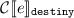, takes an expression e and a meta-variable $$\mathtt {destiny}$$ which holds the promise that the current task should fulfil, and produces an expression in the target language.

Futures are translated to promises, and most other expressions are translated homomorphically. The constructs where something interesting happens are $$\mathtt {async}$$, $$\mathtt {forward}$$ and future chaining; these constructs adopt a common pattern implemented using a two parameter lambda abstraction: the first parameter, variable $$\mathtt {destiny}'$$, is the promise to be fulfilled and the second parameter is the value that fulfils the promise. The best illustration of how $$\mathtt {forward}$$ behaves differently from a regular asynchronous call is the difference in the rules for $$\mathtt {async}~e$$ and the optimised rule for $$\mathtt {forward}\,(\mathtt {async}~e)$$. The translation of $$\mathtt {async}~e$$ creates a new promise to store e’s result value, whereas the translation of $$\mathtt {forward}\,(\mathtt {async}~e)$$ reuses the promise from the context, namely the one passed in via the $$\mathtt {destiny}$$ variable.

The compilation of configurations, denoted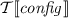, translates configurations from the source language to the target language. For example, the compilation of the source configuration $$( fut _{f} )\ ( task _{ f } \ \mathtt {forward} \, (\mathtt {async}~ e))$$ compiles into:The optimised compilation of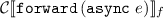is: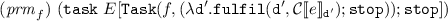For comparison, the base compilation gives: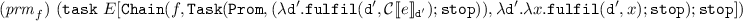Types and typing rules are compiled inductively (Fig. 7). The following lemmas guarantee that the compilation strategy does not produce broken target code and state the correctness of the translation.

### 4.1 Correctness

The correctness of the translation is proven in a number of steps.

The first step involves converting the reduction rules to a labelled transition system where communication via futures is made explicit. This involves splitting several rules involving multiple primitive configurations on the left-hand side to involve single configurations, and labelling the values going into and out of futures. For example, $$( task _{ f } \ v)\ ( fut _{f} )\rightarrow ( fut _{ f }\ v )$$ is replaced by the two rules:

$$( task _{ f } \ v)\xrightarrow {\overline{f\downarrow v}}\epsilon \qquad ( fut _{f} )\xrightarrow {f\downarrow v} ( fut _{ f }\ v )$$

The other rules introduced are:

$$( fut _{ f }\ v ) \xrightarrow {f\uparrow v} ( fut _{ f }\ v ) \qquad ( task _{ f } \ E[\mathtt {get}\, h]) \xrightarrow {\overline{h\uparrow v}} ( task _{ f } \ E[v])$$
$$( chain _{g} \ f \ e) \xrightarrow {\overline{f\uparrow v}} ( task _{ g } \ e[v/x])$$

Label $$f\downarrow v$$ captures a value being written to a future, and label $$f\uparrow v$$ captures a value being read from a future, both from the future’s perspective. Labels $$\overline{f\downarrow v}$$ and $$\overline{f\uparrow v}$$ are the duals from the perspective of the remainder of the configuration. The remainder of the rules are labelled with $$\tau$$ to indicate that no observable behaviour occurs. The same pattern is applied to the target language.

It is important to note that the values in the labels of the source language are the compiled values, while the values in the labels of the target language remain the same.Footnote 4 This is needed so that labelled values such as lambda abstraction match during the bisimulation game.

The composition rules are adapted to propagate or match labels in the standard way. For instance, the rule for matching labels in parallel configurations is: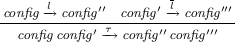The following theorems capture correctness of the translation.

### Theorem 1

If $$\varGamma \vdash config _{} \ \textit{ok} {}$$, then.

### Theorem 2

If $$\varGamma \vdash config _{} \ \textit{ok} {}$$, then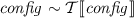.

The first theorem states that translating well-typed configurations results in well-typed configurations. The second theorem states that any well-typed configuration in the source language is bisimilar to its translation. The precise notion of bisimilarity used is bisimilarity up-to expansion . This notion of bisimilarity compresses the administrative, unobservable transitions introduced by the translation.

The proof involves taking each derivation rule in the adapted semantics for the source calculus (described above) and showing that each source configuration is bisimilar to its translation. This is straightforward for the base cases, because tasks are deterministic in both source and target languages, and at most two unobservable transitions are introduced by the translation. To handle the parallel composition of configurations, bisimulation is shown to be compositional, meaning that if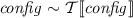and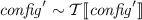, then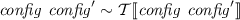; now by definition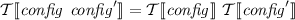, hence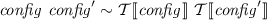.

## 5 Experiments

We benchmarked the implementation of $$\mathtt {forward}$$ by comparing it against the blocking pattern $$\mathtt{get}$$-and-$$\mathtt{return}$$ and an implementation that uses the $$\mathtt{await}$$-and-$$\mathtt{get}$$ (both described in Sect. 1). The micro-benchmark used is a variant of the broker pattern with 4 workers, compiled with aggressive optimisations ($$\mathtt{-O3}$$). We report the average time (wall clock) and memory consumption of 5 runs of this micro-benchmark under different workloads (Fig. 8). The processing of each message sent involves complex nested loops with quadratic complexity (in the Workload value) written in such a way to avoid the compiler optimising them away — the higher the workload, the higher the probability that the $$\mathtt{Broker}$$ actor blocks or awaits in the non-$$\mathtt {forward}$$ implementations.

The performance results (Fig. 8) show that the $$\mathtt{forward}$$ version is always faster than the $$\mathtt{get}$$-and-$$\mathtt{return}$$ and $$\mathtt{await}$$-and-$$\mathtt{get}$$ version. In the first case, this is expected as blocking prevents the $$\mathtt{Broker}$$ actor from processing messages, while the $$\mathtt{forward}$$ version does not block. In the second case, we also expected the $$\mathtt{forward}$$ version to be faster than the $$\mathtt{await}$$-and-$$\mathtt{get}$$: this is due to the overhead of the context switching operation performed on each $$\mathtt{await}$$ statement.

The $$\mathtt{forward}$$ version consumes the least amount of memory, while the $$\mathtt{await}$$-and-$$\mathtt{get}$$ version consumes the most (Fig. 8). This is expected: $$\mathtt {forward}$$ creates one fewer future per message sent than the other two versions; the $$\mathtt{await}$$-and-$$\mathtt{get}$$ version has around 5 times more overhead than the $$\mathtt{forward}$$ implementation, as it needs to save the context (stack) whenever a future cannot immediately be fulfilled.

Threats to Validity. The experiments use a microbenchmark, which provides useful information but is not as comprehensive as a case study would be.

## 6 Related Work

Baker discovered futures in 1977 ; later Liskov introduced promises to Argus . Around the same time, Halstead introduced implicit futures in Multilisp . Implicit futures do not appear as a first-class construct in the programming language at either the term or type level, as they do in our work.

The forward construct was introduced in earlier work , in the formalisation of an extension to the active object-based language Creol . The main differences with our work are: our core calculus is much smaller, based on tasks rather than active objects; our calculus includes closures, which complicate the type system, and future chaining; we defined a compilation strategy for forward, and benchmark its implementation.

Caromel et al.  formalise an active object language that transparently handles futures, prove determinism of the language using concepts similar to weak bisimulation, and provide an implementation . In contrast, our work uses a task-based formalism built on top of the lambda calculus and uses futures explicitly. It is not clear whether forward can be used in conjunction with transparent futures.

Proving semantics preservation of whole programs is not a new idea [17,18,19,20,21,22,23]. We highlight the work from Lochbihler, who added a new phase to the verified, machine-checked Jinja compiler  that proves that the translation from multi-threaded Java programs to Java bytecode is semantics preserving, using a delay bisimulation. In contrast, our work uses an on-paper proof using weak bisimilarity up-to expansion, proving that the compilation strategy preserves the semantics of the high-level language.

Ábrahám et al.  present an extension of the Creol language with promises. The type system uses linear types to track the use of the write capability (fulfilment) of promises to ensure that they are fulfilled precisely once. In contrast to the present work, their type system is significantly more complex, and no forward operation is present. Curiously, Encore supports linear types, though lacks promises and hence does not use linear types to keep promises under control.

Niehren et al.  present a lambda calculus extended with futures (which are really promises). Their calculus explores the expressiveness of programming with promises, by using them to express channels, semaphores, and ports. They also present a linear type system that ensures that promises are assigned only once.

## 7 Conclusion

One key difference between futures, futures with forward and promises is that the responsibility to fulfil a future cannot be delegated. The $$\mathtt {forward}$$ construct allows such delegation, although only of the implicit future receiving the result of some method call, while promises allow arbitrary delegation of responsibility. This paper presented a formal calculus capturing the $$\mathtt {forward}$$ construct, which retains the static fulfilment guarantees of futures. A translation of the source calculus into a target calculus based on promises was provided and proven to be semantics preserving. This translation models how $$\mathtt {forward}$$ is implemented in the Encore compiler. Microbenchmarks demonstrated that $$\mathtt {forward}$$ improves performance in terms of speed and memory overhead compared to two alternative implementations in the Encore language.